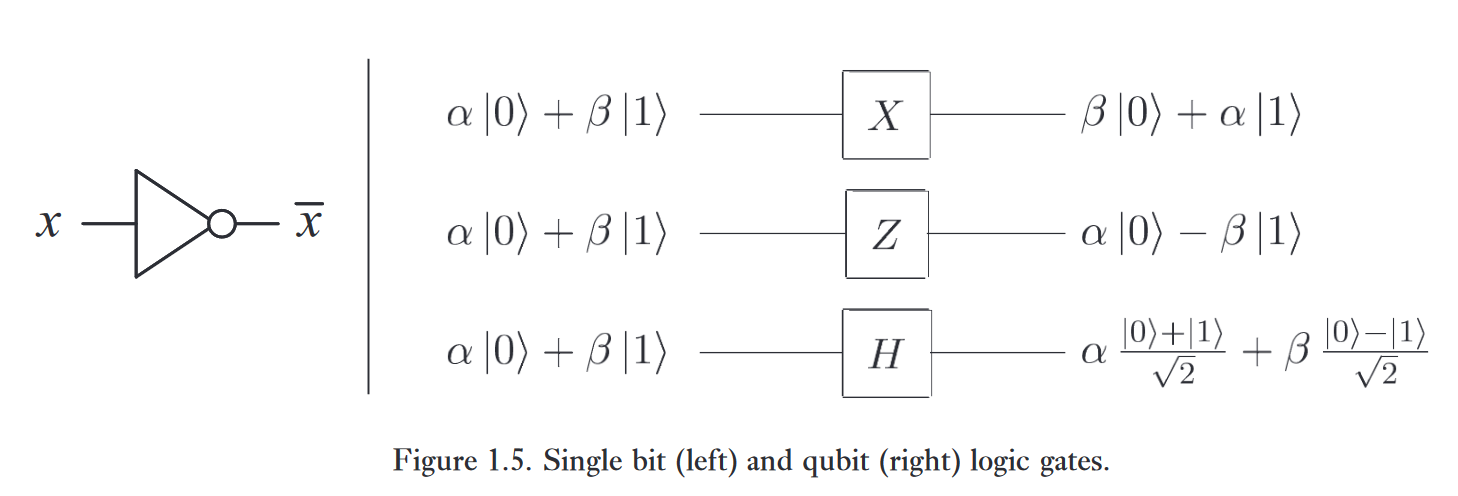【REPO】量子计算与量子信息笔记

# 2.Qubit

## 2.1.单个量子比特

$|\psi\rangle = \alpha|0\rangle+\beta|1\rangle$

$\frac{1}{\sqrt2} |0\rangle+\frac{1}{\sqrt2}|1\rangle$

$|+\rangle = \frac{1}{\sqrt2} |0\rangle+\frac{1}{\sqrt2}|1\rangle$

## 2.2.多个量子比特

00，01，10，11

$|00\rangle,|01\rangle,|10\rangle,|11\rangle$

$|\psi\rangle = \alpha_{00}|00\rangle+\alpha_{01}|01\rangle+\alpha_{10}|10\rangle+\alpha_{11}|11\rangle$

$\sum_{x\in\{0,1\}^2}|\alpha_x|^2=1$

$|\alpha_{00}|^2+|\alpha_{01}|^2$

$|\psi'\rangle = \frac{\alpha_{00}|00\rangle+\alpha_{01}|01\rangle}{\sqrt{|\alpha_{00}|^2+|\alpha_{01}|^2}}$

## 2.3.单量子比特门

single qubit gates

$X = \begin{bmatrix} 0 & 1 \\ 1 & 0 \end{bmatrix}$

$X\begin{bmatrix} \alpha \\ \beta \end{bmatrix} = \begin{bmatrix} \beta \\ \alpha \end{bmatrix}$

$U^\dagger U = I$

• Z门：

$Z = \begin{bmatrix} 1 & 0 \\ 0 & -1 \end{bmatrix}$

$|0\rangle$ 保持不变，$|1\rangle$ 变为 $-|1\rangle$

$H = \frac{1}{\sqrt{2}}\begin{bmatrix} 1 & 1 \\ 1 & -1 \end{bmatrix}$2.4.1.量子不可克隆

# 参考

 Quantum Computation and Quantum Information